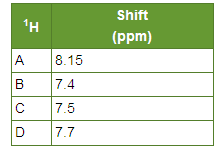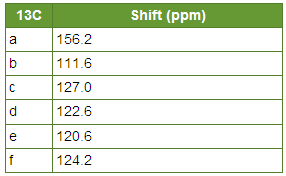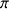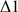# Heteronuclear Correlations

$$\newcommand{\vecs}{\overset { \rightharpoonup} {\mathbf{#1}} }$$ $$\newcommand{\vecd}{\overset{-\!-\!\rightharpoonup}{\vphantom{a}\smash {#1}}}$$$$\newcommand{\id}{\mathrm{id}}$$ $$\newcommand{\Span}{\mathrm{span}}$$ $$\newcommand{\kernel}{\mathrm{null}\,}$$ $$\newcommand{\range}{\mathrm{range}\,}$$ $$\newcommand{\RealPart}{\mathrm{Re}}$$ $$\newcommand{\ImaginaryPart}{\mathrm{Im}}$$ $$\newcommand{\Argument}{\mathrm{Arg}}$$ $$\newcommand{\norm}{\| #1 \|}$$ $$\newcommand{\inner}{\langle #1, #2 \rangle}$$ $$\newcommand{\Span}{\mathrm{span}}$$ $$\newcommand{\id}{\mathrm{id}}$$ $$\newcommand{\Span}{\mathrm{span}}$$ $$\newcommand{\kernel}{\mathrm{null}\,}$$ $$\newcommand{\range}{\mathrm{range}\,}$$ $$\newcommand{\RealPart}{\mathrm{Re}}$$ $$\newcommand{\ImaginaryPart}{\mathrm{Im}}$$ $$\newcommand{\Argument}{\mathrm{Arg}}$$ $$\newcommand{\norm}{\| #1 \|}$$ $$\newcommand{\inner}{\langle #1, #2 \rangle}$$ $$\newcommand{\Span}{\mathrm{span}}$$$$\newcommand{\AA}{\unicode[.8,0]{x212B}}$$

The heteronuclear correlation of chemical shifts by scalar coupling derives from the existence of heteronuclear scalar coupling all owing a magnetization transfer from the more sensitive nucleus (1H) toward the less sensitive nucleus (13C). The model experiment is the correlation δ(13C)/ δ(1H) which uses the large direct coupling 1J(13C-1H) comprised between more 100 Hz and 200Hz and which allows to correlate the signals from a proton to that of a 13C to which it is bound (5).

## The XHCORR sequence

This sequence allows to correlate the signals of the 1H and of the 13C bound to each other (13). Spectrum 11 (XHCORR), shows the correlations for the dihydrofuran (Fig. 31) between bound carbons and protons. The chemical shifts of the protons of these molecules are in the table 2, those of the carbon are in the table 3.Table 2: DBF protons chemical shift.Table 3: DBF carbons chemical shiftSpectrum: XHCORR of the DBF

Within the XHCORR pulse sequence (Fig. 25), transverse magnetization is caused by aimpulsion which is evolving during the t1 period. Theimpulsion (13C), located in the middle of this period refocuses the heteronuclear couplings.

The optimization of theanddelays allows the selection of the long range heteronuclear couplings, this means that instead of seeing the correlation between 13C and protons directly bound, we favor the appearance of the correlations spots between 13C and non bound protons (HNB).

For example, for a coupling constant J=10 Hz then=50 ms and=33 ms.

## The COLOC sequence (COrrelation via LOng range Coupling)

The sequence which is used (Fig. 26) allows generally a good correlation between the quaternary carbons and the neighbouring protons. On the spectrum 12 (COLOC), the correlation between the quaternary carbon (13C = δ = 156. 2 ppm) and its vicinal proton (δ = 7.7 ppm) is named Cq. However, it does not give good results for long range correlations involving protonated carbons (14). This observation led to write a pulse sequence named XC ORFE (X nucleus proton CORrelation with Fixed Evolution time). This one is very closed to the COLOC sequence.Spectrum 12: COLOC of DBF

## Heteronuclear 2D J-resolved Pulse Sequence

In this experiment we observe the coupling J between the X nuclei (13C, 15N, 31P ...etc ) and the protons which are bound to them (Fig. 27). A coupled normal 1D spectrum XH may be very difficult to explain. This is due to the intercrossing of the multiplets (12). For simplifying the spectra, we will spread the coupling data in the Fl dimension and the informations arising from the chemical shifts in the F2 dimension.